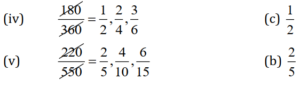# Ncert Class 6 Math Fractions Exercise 7.3

Fractions Class 6 Ex. 7.3

New Ncert Class 6, Chapter 7 Math Free Solution.

Exercise 7.3

Question 1 :- Write the fractions. Are all these fractions equivalent?

(a)(b)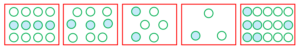Solution 1:-

(a) 1/2,  2/4,  3/6,  4/8;

Yes, all of these fractions are equivalent.

(b) 4/12,  3/9, 2/6,  1/3,  6/15;

No, all of these fractions are not equivalent.

Question 2 :- Write the fractions and pair-up the equivalent-fractions from each rows.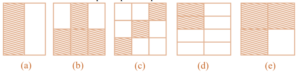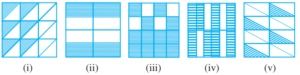Solution 2 :-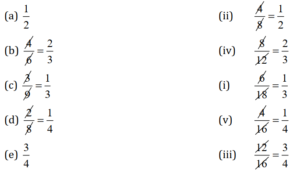The following pairs fractions;  represent the equivalent fractions.
(a) and (ii) = 1/2;
(b) and (iv) = 2/3;
(c) and (i) = 1/3;
(d) and (v) = 1/4;
(e) and (iii) = 3/4;

Question 3 :- Replace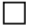in each of the following by the correct number: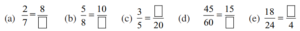Solution 3 :-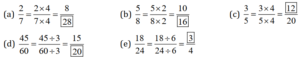Question 4 :- Find, the equivalent-fraction of 3/5 having :-
(a) denominator 20
(b) numerator 9
(c) denominator 30
(d) numerator 27

Solution 4:-
(a) Here, we require denominator 20.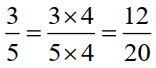∴ The required fraction is 12/20

(b) Here, we required numerator 9.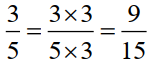∴ The required fraction is 9/15.

(c) Here, we required denominator 30.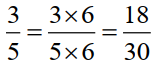∴ The required fraction is 18/30.

(d) Here, we required numerator 27.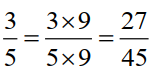∴ The required fraction is 27/45.

Question 5 :- Find, the equivalent-fraction of 36/48 withs :-
(a) numerator 9
(b) denominator 4

Solution 5:-
(a) Given that numerator = 9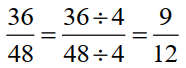So, the equivalent fraction is 9/12.

(b) Given that denominator = 4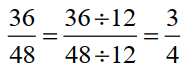So, the equivalent fraction is 3/4 .

Question 6 :- Check, whether the given fractionss are equivalent :-

(a) 5/9,  30/54;

(b) 3/10,  12/50;

(c) 7/13,  5/11;

Solution 6:-
(a) 5/9 and 30/54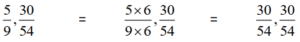So; 5/9 and 30/54 are equivalent fractions.

(b) 3/10 and 12/50;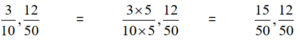So; 3/10 and 12/50 are not equivalent fractions.

(c) 7/13 and 5/11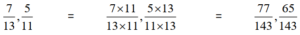So; 5/11 and 7/13 do not have the same fractional value.

Question 7 :- Reduce the following fraction into a simplest form :-

(a) 48/60;

(b) 150/60;

(c) 84/98;

(d) 12/52;

(e) 7/28;

Solution 7:-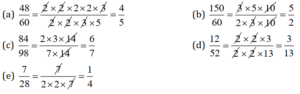Question 8 :- Ramesh had 20 pencil, Sheelu had 50 pencil and Jamaal had 80 pencil. After 4 months, Ramesh used-up 10 pencil, Sheelu used-up 25 pencil and Jamaal used-up 40 pencil. What fraction did each used up? Check if each has used up an equal fraction of her/his pencils.

Solution 8:-
Out of a total of 20, Ramesh used 10 pencils.

Fraction = 10/20 = 1/2;

Out of a total of 50, Sheelu used 25 pencils.

Fraction = 25/50 = 1/2;

Out of a total of 80, Jamaal used 40 pencils.

Fraction = 40/80 = 1/2;

Yes, each has used up an equal fractions, which is  1/2.

Question 9 :- Match the equivalent-fraction and write two more for each.

(i)  250/400              (a) 2/3;

(ii) 180/200               (b) 2/5;

(iii) 660/990              (c) 1/2;

(iv) 180/360               (d) 5/8;

(v) 7/28                       (e) 7/28;
Solution 9:-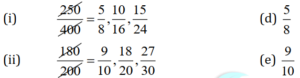(iii)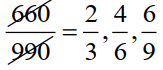(a) 2/3;